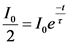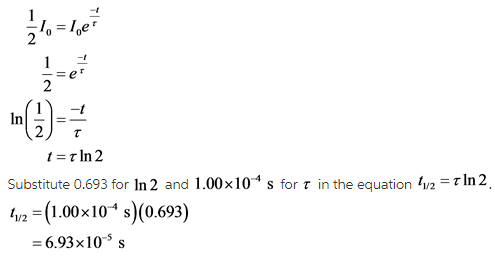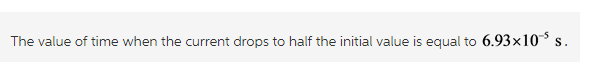# At t =0s, the current in the circuit in the figure is I0. At what time is the current 12I0?

At t =0s, the current in the circuit in the figure is I0. At what time is the current 12I0?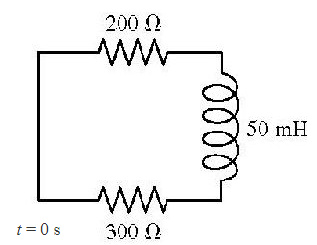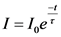Substitute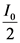for I in above equation as follows: